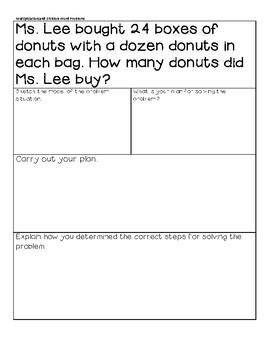DID YOU KNOW:
Seamlessly assign resources as digital activities

Learn how in 5 minutes with a tutorial resource. Try it Now

Learn More# MiniLesson: Multiplication and Division Review w/ Practice Word ProblemsGrade Levels
3rd - 5th
Subjects
Standards
Resource Type
Formats Included
• PDF
• Compatible withActivities
Pages
11 pages
Compatible with Easel Activities
Create an interactive version of this PDF students can complete on any device. Easel is free to use! Learn more.

### Description

Objective: Students will be able to solve one- and two- step problems using involving multiplication and division, correctly determining the operation and steps to match with visual representations of the problem situations.

Mini Lesson components include:

Objective

Vocabulary

Guiding Questions

Differentiation Ideas

Materials list

Answer Key

and Implementation

Also includes, strip diagram and representation cards and 5 word problems for partners to review and practice.

4th grade Math TEXAS ESSENTIAL KNOWLEDGE & SKILL

4.4H solve with fluency one- and two-step problems involving multiplication and division, including interpreting remainders.

Total Pages
11 pages
Answer Key
Included
Teaching Duration
30 minutes
Report this Resource to TpT
Reported resources will be reviewed by our team. Report this resource to let us know if this resource violates TpT’s content guidelines.

### Standards

to see state-specific standards (only available in the US).
Model with mathematics. Mathematically proficient students can apply the mathematics they know to solve problems arising in everyday life, society, and the workplace. In early grades, this might be as simple as writing an addition equation to describe a situation. In middle grades, a student might apply proportional reasoning to plan a school event or analyze a problem in the community. By high school, a student might use geometry to solve a design problem or use a function to describe how one quantity of interest depends on another. Mathematically proficient students who can apply what they know are comfortable making assumptions and approximations to simplify a complicated situation, realizing that these may need revision later. They are able to identify important quantities in a practical situation and map their relationships using such tools as diagrams, two-way tables, graphs, flowcharts and formulas. They can analyze those relationships mathematically to draw conclusions. They routinely interpret their mathematical results in the context of the situation and reflect on whether the results make sense, possibly improving the model if it has not served its purpose.
Make sense of problems and persevere in solving them. Mathematically proficient students start by explaining to themselves the meaning of a problem and looking for entry points to its solution. They analyze givens, constraints, relationships, and goals. They make conjectures about the form and meaning of the solution and plan a solution pathway rather than simply jumping into a solution attempt. They consider analogous problems, and try special cases and simpler forms of the original problem in order to gain insight into its solution. They monitor and evaluate their progress and change course if necessary. Older students might, depending on the context of the problem, transform algebraic expressions or change the viewing window on their graphing calculator to get the information they need. Mathematically proficient students can explain correspondences between equations, verbal descriptions, tables, and graphs or draw diagrams of important features and relationships, graph data, and search for regularity or trends. Younger students might rely on using concrete objects or pictures to help conceptualize and solve a problem. Mathematically proficient students check their answers to problems using a different method, and they continually ask themselves, "Does this make sense?" They can understand the approaches of others to solving complex problems and identify correspondences between different approaches.
Solve multistep word problems posed with whole numbers and having whole-number answers using the four operations, including problems in which remainders must be interpreted. Represent these problems using equations with a letter standing for the unknown quantity. Assess the reasonableness of answers using mental computation and estimation strategies including rounding.
Multiply or divide to solve word problems involving multiplicative comparison, e.g., by using drawings and equations with a symbol for the unknown number to represent the problem, distinguishing multiplicative comparison from additive comparison.

### Questions & Answers

Teachers Pay Teachers is an online marketplace where teachers buy and sell original educational materials.

More About Us